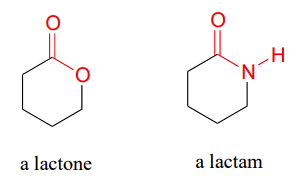# 21.0: Chapter Objectives and Introduction to Carboxylic Acid Derivatives

##### Objectives
1. fulfill all of the detailed objectives listed under each individual section.
2. design multi‑step syntheses in which the reactions introduced in this chapter are used in conjunction with any of the reactions described in previous chapters.
3. solve road‑map problems that require a knowledge of the chemistry of carboxylic acid derivatives.
4. define, and use in context, any of the key terms introduced in this chapter.

After completing this section, you should be able to

1. identify, and give an example of
1. an acid halide.
2. an acid anhydride.
3. an ester.
4. an amide.
2. write the general form of a nucleophilic acyl substitution reaction.
##### Key Terms

Make certain that you can define, and use in context, the key terms below.

• acyl derivative
• nucleophilic acyl substitution reaction

## Carboxylic Acid Derivatives

Carboxylic acid derivatives are formed when the hydroxyl group of a carboxylic acid is replaced by some other group, $$\ce{Y}$$, such that it can be hydrolyzed back to the acid.These compounds are also called the acyl derivatives of a carboxylic acid because they usually contain an acyl group bonded to a substituent which can act as a leaving group in a nucleophilic acyl substitution reaction.Although there are many types of carboxylic acid derivatives known, this chapter focuses on six: acid halides (acyl halides), anhydrides, esters, amides, thioesters, and acylphosphates.Cyclic esters and amides are referred to as lactones and lactams, respectively.Nitriles, $$\ce{RC \equiv N}$$, discussed in the last chapter, are often are considered to be carboxylic acid derivatives, even though the acyl group is not present, because hydrolysis of nitriles leads to carboxylic acids.## Nucleophilic Acyl Substitution Reaction

The main type of reaction for carboxylic acid derivatives is called nucleophilic acyl substitution. This reaction involves the replacement of the $$\ce{Y}$$ group by attack of a nucleophile $$\ce{Nu}^\ominus$$ at the carbonyl carbon with subsequent cleavage of the $$\ce{C-Y}$$ bond.## Physical Properties of Carboxylic Acid Derivatives

The intermolecular forces of the respective acyl group combine with the size and structure of the Y-group to determine the physical properties of the the carboxylic acid derivatives as shown in the summary below.